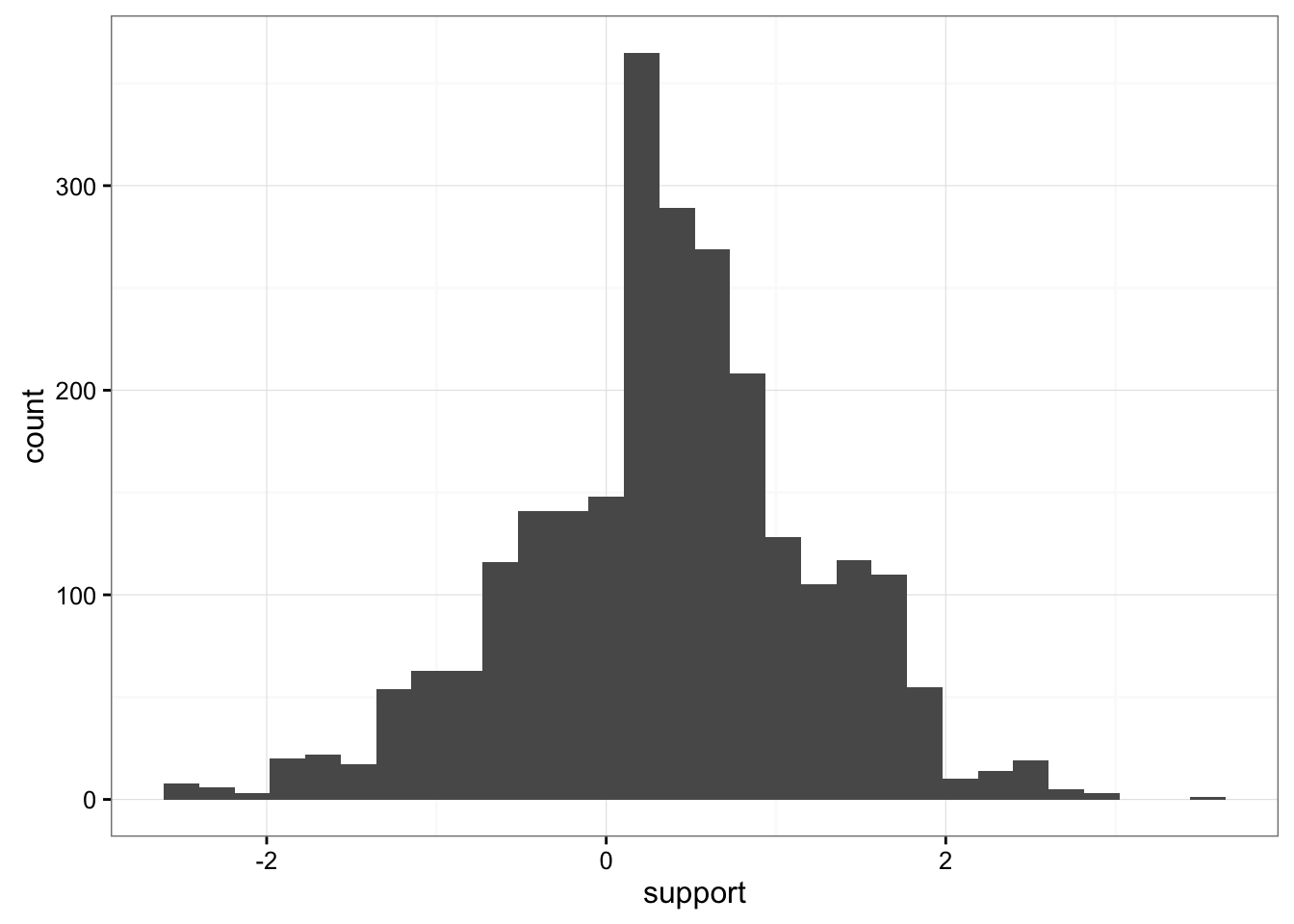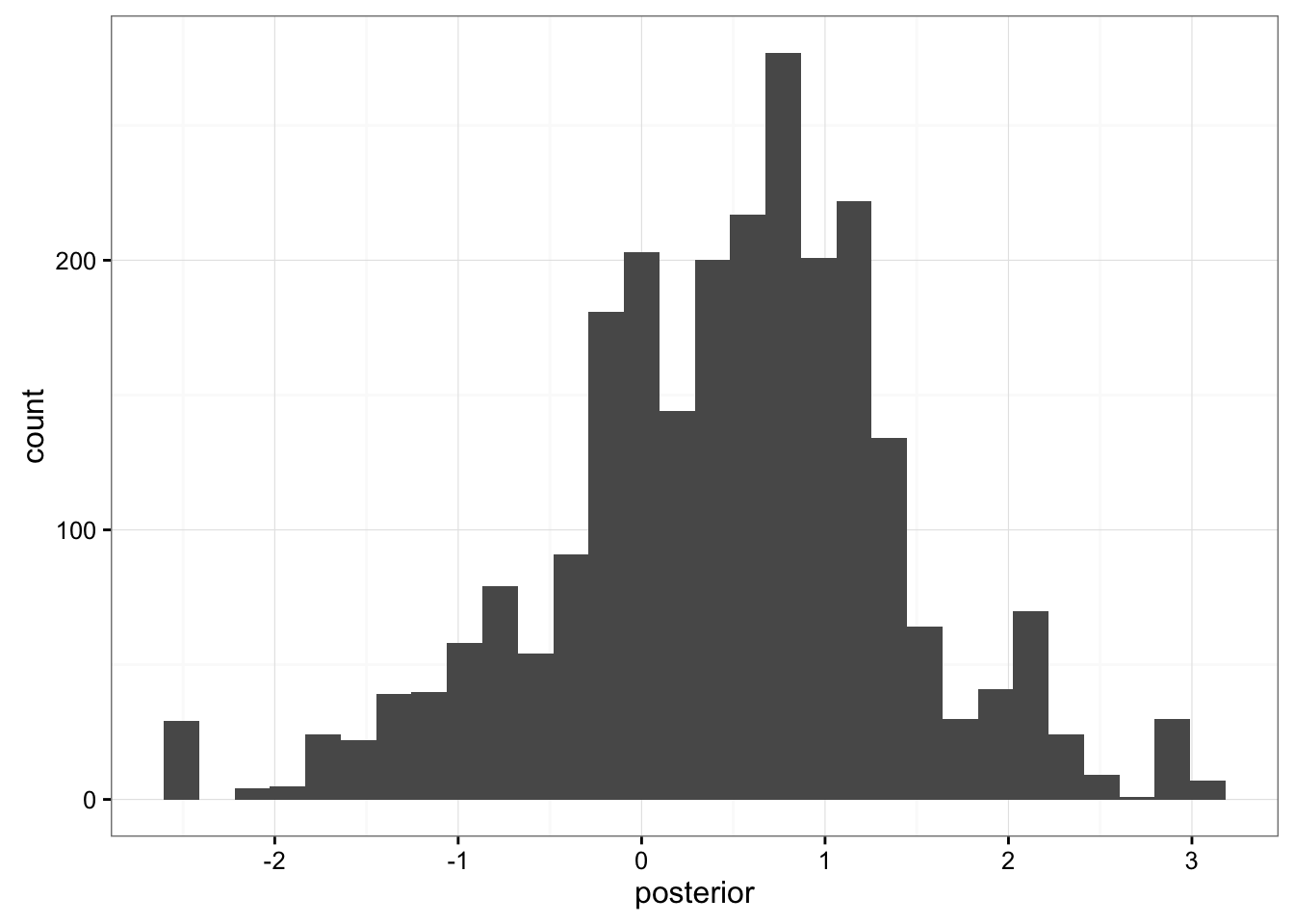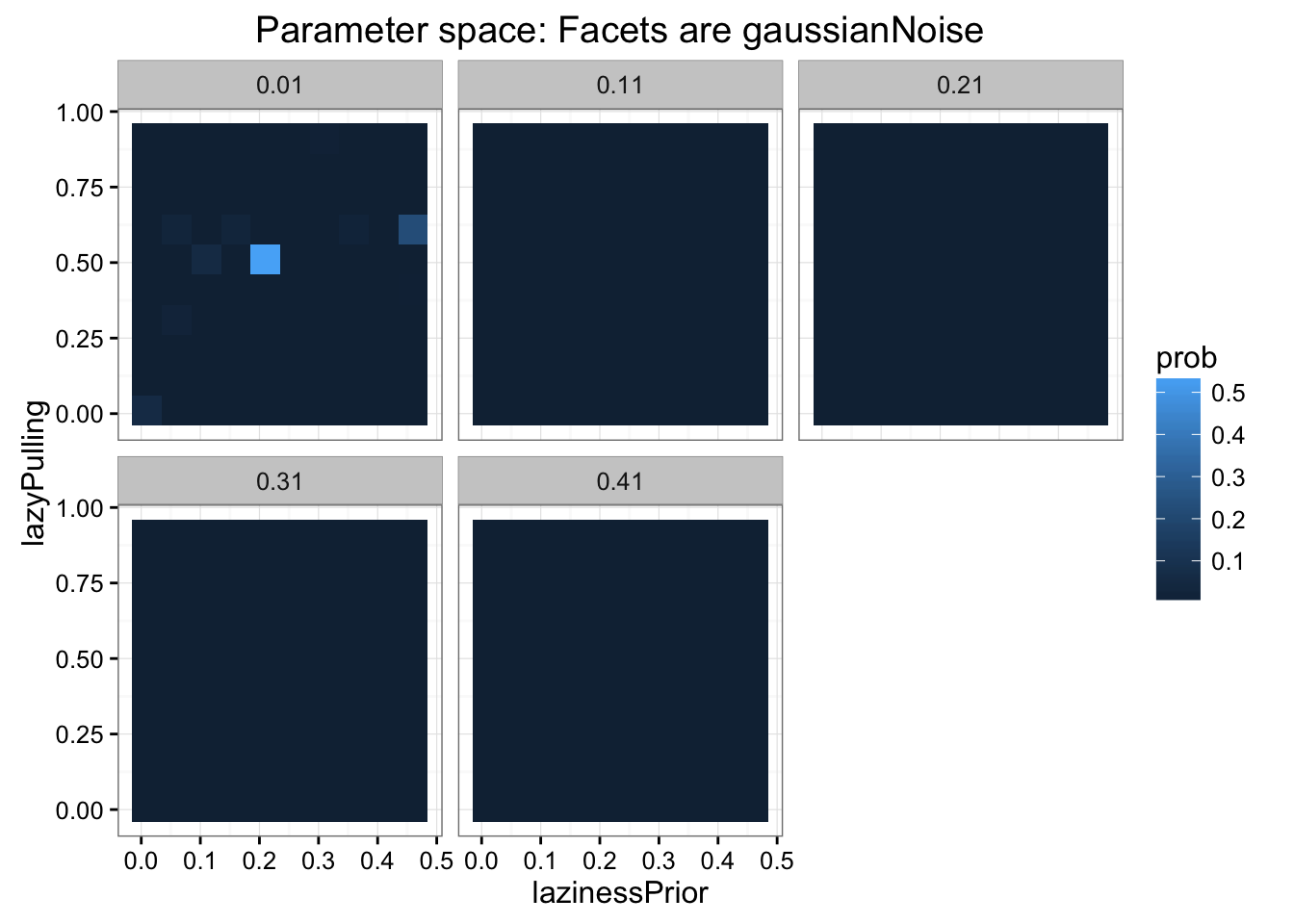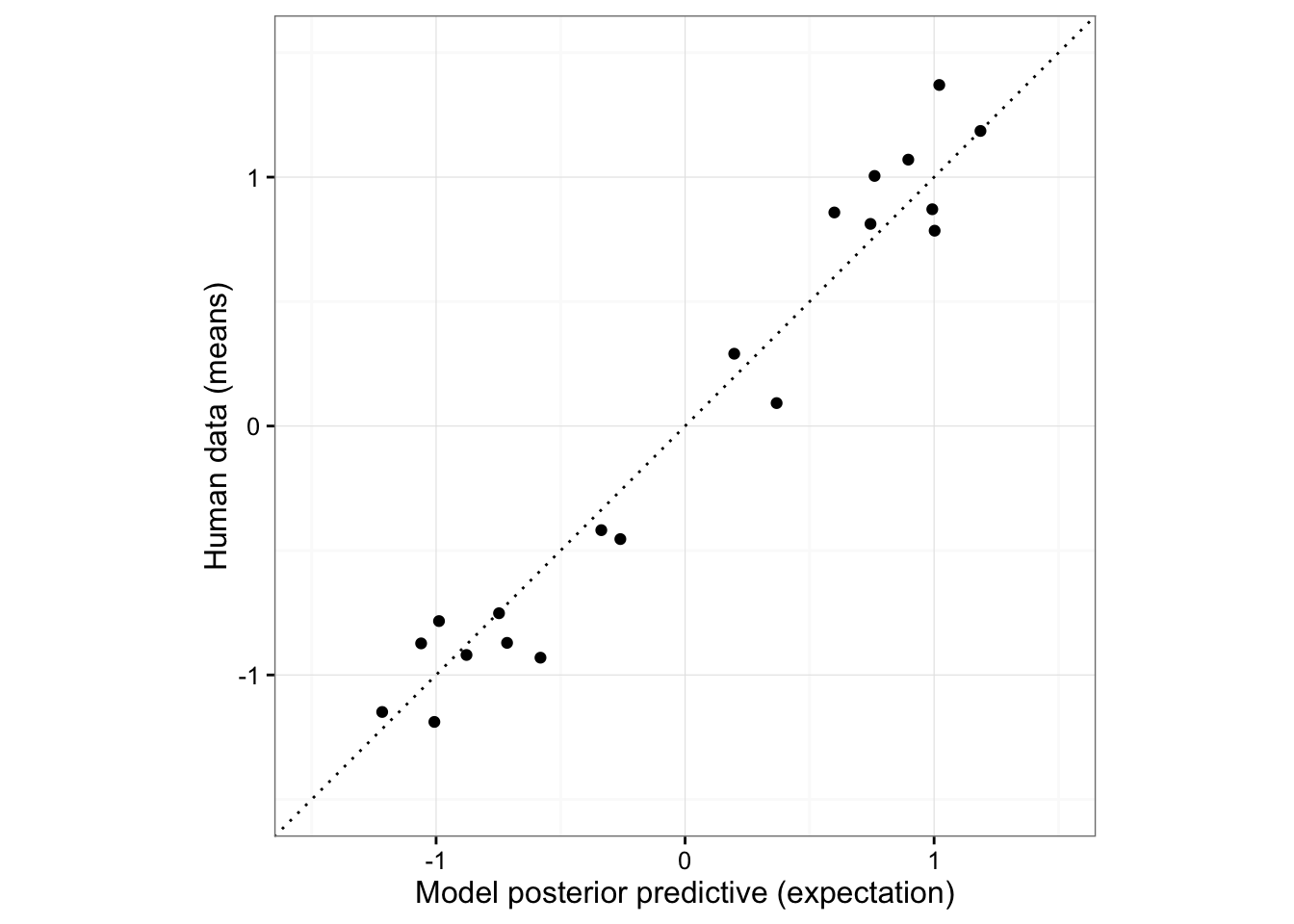``````library(knitr)
knitr::opts_chunk\$set(fig.crop = F,echo=T,
warning=F, cache=F,
message=F, sanitize = T)

library(rwebppl)``````
``## local webppl exists: master v0.9.2-838292c /usr/local/lib/node_modules/webppl``
``````library(dplyr)
library(coda)
estimate_mode <- function(s) {
d <- density(s)
return(d\$x[which.max(d\$y)])
}
hdi_upper<- function(s){
m <- HPDinterval(mcmc(s))
return(m["var1","upper"])
}
hdi_lower<- function(s){
m <- HPDinterval(mcmc(s))
return(m["var1","lower"])
}``````

## RWebPPL

This is an example of using RWebPPL (inside an R Markdown document) to run Bayesian data analysis on cognitive models using WebPPL. Those familiar with R might find the RWebPPL package useful. More information about RWebPPL can be found here.

## Basic usage of RWebPPL

``````towModel <- '
var options = {method: "MCMC", samples: 2500}

var lazinessPrior = 0.3;
var lazyPulling = 0.5;

var model = function() {

var strength = mem(function(person){
return gaussian(0, 1)
})
var lazy = function(person){
return flip(lazinessPrior)
}
var pulling = function(person) {
return lazy(person) ?
strength(person) * lazyPulling :
strength(person)
}
var totalPulling = function(team){return sum(map(pulling, team)) }
var winner = function(team1, team2){
totalPulling(team1) > totalPulling(team2) ? team1 : team2
}
var beat = function(team1,team2){winner(team1,team2) == team1}

condition(beat(["bob", "mary"], ["tom", "sue"]))

return strength("bob")
}

var posterior = Infer(options, model)
display("Bobs strength, given that he and Mary beat Tom and Sue")
display("Expected value = " + expectation(posterior))
posterior
'
posterior <- webppl(towModel)``````

The results of the WebPPL program are captured in the output, automatically converted into an R data frame.

``head(posterior)``
``````##      support   prob
## 1  0.2319662 0.0060
## 2  0.1339641 0.0028
## 3  0.2500428 0.0012
## 4 -0.8041205 0.0004
## 5  1.5574405 0.0012
## 6  0.5031501 0.0024``````

RWebPPL ships with a helper function called `get_samples()`, which will allow you to easily convert the default output of WebPPL (which is as a histogram) into samples, which allows for easy interplay with R visualization programs (e.g., `ggplot()`).

``````posterior.samples <- get_samples(posterior, 2500)
``````##       support
## 1   0.2319662
## 1.1 0.2319662
## 1.2 0.2319662
## 1.3 0.2319662
## 1.4 0.2319662
## 1.5 0.2319662``````
``````ggplot(posterior.samples, aes ( x = support ) )+
geom_histogram()``````### Passing data to WebPPL

Using RWebPPL, you can pass data from R to WebPPL.

Let’s modify the tug of war model to define `lazinessPrior` and `lazyPulling` with a variable that we’ll pass in.

``````towModelNoData <- '
var options = {method: "MCMC", samples: 2500}

var lazinessPrior = dataFromR.lazyPrior;
var lazyPulling = dataFromR.lazyPulling;

var model = function() {

var strength = mem(function(person){
return gaussian(0, 1)
})
var lazy = function(person){
return flip(lazinessPrior)
}
var pulling = function(person) {
return lazy(person) ?
strength(person) * lazyPulling :
strength(person)
}
var totalPulling = function(team){return sum(map(pulling, team)) }
var winner = function(team1, team2){
totalPulling(team1) > totalPulling(team2) ? team1 : team2
}
var beat = function(team1,team2){winner(team1,team2) == team1}

condition(beat(["bob", "mary"], ["tom", "sue"]))

return strength("bob")
}

var posterior = Infer(options, model)
display("Bobs strength, given that he and Mary beat Tom and Sue")
display("Expected value = " + expectation(posterior))
posterior
'``````

To see what your R data structure will look like in WebPPL, you can use the `jsonlite` package (loaded via `library(jsonlite)`) and pass your data structure (or, some subset) through the function `toJSON()`.

``````library(jsonlite)
dataToWebPPL <- data.frame(lazyPrior = 0.3, lazyPulling = 0.5)
toJSON(dataToWebPPL, pretty = T)``````
``````## [
##   {
##     "lazyPrior": 0.3,
##     "lazyPulling": 0.5
##   }
## ]``````

Note that data.frames get converted into arrays of objects, and lists get converted into objects of lists.

All we need to tell RWebPPL is the data we want to pass to WebPPL (`data = ...`) and what the data structure will be labeled inside WebPPL (`data_var = ...`).

``````webppl(towModelNoData,
data = dataToWebPPL,
data_var = "dataFromR") %>%
get_samples(., 2500) %>%
ggplot(., aes ( x = support ) )+
geom_density()``````

### Specifying `Infer` options in R

When engaging in Bayesian data analysis of rich cognitive models, we are often running `Infer` in the outer loop (for the data analysis model) and `Infer` in the innter loop (for the cognitive model). The outer loop `Infer` will often be computationally challenging. Thus, it is often useful to be able to try a number of different inference strategies (via, `{method: ...}` in the inference options). To faciliate this, RWebPPL allows you to specify inference options in R. You just need to tell RWebPPL what model (spec., what thunk) to do inference over (`model_var = ...`), and the options (`inference_opts = ...`).

Here, we will demonstrate this on the Tug of War cognitive model.

``````towModelNoDataNoInfer <- '
var lazinessPrior = dataFromR.lazyPrior;
var lazyPulling = dataFromR.lazyPulling;

var model = function() {
var strength = mem(function(person){
return gaussian(0, 1)
})
var lazy = function(person){
return flip(lazinessPrior)
}
var pulling = function(person) {
return lazy(person) ?
strength(person) * lazyPulling :
strength(person)
}
var totalPulling = function(team){return sum(map(pulling, team)) }
var winner = function(team1, team2){
totalPulling(team1) > totalPulling(team2) ? team1 : team2
}
var beat = function(team1,team2){winner(team1,team2) == team1}

condition(beat(["bob", "mary"], ["tom", "sue"]))

return strength("bob")
}
'``````
``````posterior <- webppl(towModelNoDataNoInfer,
data = dataToWebPPL,
data_var = "dataFromR",
inference_opts = list(
method = "MCMC",
samples = 2500),
model_var = "model")

``````##      support   prob
## 1  0.1959981 0.0020
## 2  0.2998611 0.0008
## 3  1.1064905 0.0216
## 4  0.2166167 0.0048
## 5 -1.0990272 0.0012
## 6  0.2432447 0.0040``````

Note the posterior variable in R is the result of calling `Infer()` on `model_var`.

#### Specifying output format

If you are specifying the inference options in R, you can also change the output format of the main Infer. Currently, the output formats supported are `samples` (each row of data frame is a sample), `ggmcmc` (for use with the ggmcmc R package; Note: not all functionality for this is currently supported), and `webppl` (default, webppl histogram).

``````posterior <- webppl(towModelNoDataNoInfer,
data = dataToWebPPL,
data_var = "dataFromR",
inference_opts = list(
method = "MCMC",
samples = 2500),
model_var = "model",
output_format = "samples")

``````##     support
## 1   2.05056
## 1.1 2.05056
## 1.2 2.05056
## 1.3 2.05056
## 1.4 2.05056
## 1.5 2.05056``````
``qplot(posterior)``# Bayesian Data Analysis of Tug of War model

## Tug of War data

First, we’ll load the raw tug of war data generiously supplied by Tobias Gerstenberg, from Gerstenberg & Goodman (2012).

``````df.tow <- read.csv("https://raw.githubusercontent.com/probmods/probmods2/master/assets/data/towData.csv")

``````##   id trial rating    ratingZ outcome                  pattern tournament
## 1  1     1     61  0.6093882     win      confounded evidence     single
## 2  1     2     13 -2.1501057    loss      confounded evidence     single
## 3  1     3     62  0.6668777     win strong indirect evidence     single
## 4  1     4     33 -1.0003165    loss strong indirect evidence     single
## 5  1     5     46 -0.2529536     win   weak indirect evidence     single
## 6  1     6     45 -0.3104431    loss   weak indirect evidence     single``````
``summary(df.tow)``
``````##        id           trial           rating          ratingZ
##  Min.   : 1.0   Min.   : 1.00   Min.   :  0.00   Min.   :-2.1501
##  1st Qu.: 8.0   1st Qu.: 5.75   1st Qu.: 24.00   1st Qu.:-0.8884
##  Median :15.5   Median :10.50   Median : 50.00   Median :-0.1194
##  Mean   :15.5   Mean   :10.50   Mean   : 50.65   Mean   : 0.0000
##  3rd Qu.:23.0   3rd Qu.:15.25   3rd Qu.: 79.00   3rd Qu.: 0.9549
##  Max.   :30.0   Max.   :20.00   Max.   :100.00   Max.   : 2.1041
##
##  outcome                        pattern     tournament
##  loss:300   confounded evidence     : 60   double:360
##  win :300   confounded with opponent: 60   single:240
##             confounded with partner : 60
##             diverse evidence        :120
##             round robin             : 60
##             strong indirect evidence:120
##             weak indirect evidence  :120``````
``````# we add a rounded version of the rating data for easy of inference
df.tow\$roundedRating <- round(df.tow\$ratingZ, 1)``````

What will the data look like in R?

``toJSON(head(df.tow), pretty = T)``
``````## [
##   {
##     "id": 1,
##     "trial": 1,
##     "rating": 61,
##     "ratingZ": 0.6094,
##     "outcome": "win",
##     "pattern": "confounded evidence",
##     "tournament": "single",
##     "roundedRating": 0.6
##   },
##   {
##     "id": 1,
##     "trial": 2,
##     "rating": 13,
##     "ratingZ": -2.1501,
##     "outcome": "loss",
##     "pattern": "confounded evidence",
##     "tournament": "single",
##     "roundedRating": -2.2
##   },
##   {
##     "id": 1,
##     "trial": 3,
##     "rating": 62,
##     "ratingZ": 0.6669,
##     "outcome": "win",
##     "pattern": "strong indirect evidence",
##     "tournament": "single",
##     "roundedRating": 0.7
##   },
##   {
##     "id": 1,
##     "trial": 4,
##     "rating": 33,
##     "ratingZ": -1.0003,
##     "outcome": "loss",
##     "pattern": "strong indirect evidence",
##     "tournament": "single",
##     "roundedRating": -1
##   },
##   {
##     "id": 1,
##     "trial": 5,
##     "rating": 46,
##     "ratingZ": -0.253,
##     "outcome": "win",
##     "pattern": "weak indirect evidence",
##     "tournament": "single",
##     "roundedRating": -0.3
##   },
##   {
##     "id": 1,
##     "trial": 6,
##     "rating": 45,
##     "ratingZ": -0.3104,
##     "outcome": "loss",
##     "pattern": "weak indirect evidence",
##     "tournament": "single",
##     "roundedRating": -0.3
##   }
## ]``````

Here, we see that the data frame will be converted into an array of objects. The data analysis model presented at the end of the chapter is expected a data structure like this, so we are in good shape.

Because we are writing the WebPPL model as a string, we can write the program in chunks, and use R’s `paste()` function to place it all back together again.

#### Helper functions

``````helpers <- '
var levels = function(a, lvl){ return _.uniq(_.pluck(a, lvl)) }

var outcomes = levels(towData, "outcome");
var tournaments = levels(towData, "tournament");
var patterns = {
single: levels(_.where(towData, {tournament: "single"}), "pattern"),
double: levels(_.where(towData, {tournament: "double"}), "pattern")
};

var round = function(x){
return Math.round(x*10)/10
}

var bins = map(round, _.range(-2.2, 2.2, 0.1))

// add a tiny bit of noise, and make sure every bin has at least epsilon probability
var smoothToBins = function(dist, sigma, bins){
Infer({method: "enumerate"}, function(){
var x = sample(dist);
var smoothedProbs = map(function(b){
return Number.EPSILON+
Math.exp(Gaussian({mu: x, sigma: sigma}).score(b))
}, bins)
return categorical(smoothedProbs, bins)
})
}
'``````

#### Tug of war Model (Bayes in the head)

Because we are going to do Bayesian inference over the parameters `lazyPulling` and `lazinessPrior`, we turn them into arguments of the `tugOfWarModel` function. We also abstract the match information so it’s also an argument. (Because the empirical data is collected from several different conditions, corresponding to different tournament setups.)

When you do Bayesian data analysis of Bayesian cognitive models, you have to spend a litle bit of time thinking about inference strategies in WebPPL (i.e., whatever goes in to `{method: ..., ...}`), because there will be (at least) 2 of them: the inner model (the cognitive model) and the outer model (the data analysis model).

For the inner model, you want a strategy that will give reliable results run-after-run. You will be running the model multiple times in the data analysis model and you want the differences you see across different sampled parameters (different runs of the outer model) to be attributed to the different parameter values and not intrinsic randomness in the posterior inference of the cognitive model

For this, enumeration is highly preferred. Models should be discretized when possible to allow the usage of enumeration, and multiple discretization strategies should be tried to ensure that the discretization is not driving the model behavior. For the tug-of-war model, enumeration is very expensive (because you have conditions with 3 doubles tournaments, and you have to approximate strength and lazy variables for 12 players…). Here we use rejection sampling, because the samples are samples from the true posterior and it runs reasonably quickly. Preliminary explorations suggested that 500 or 1000 samples give reasonably reliable results, but this should be tested more systematically. It’s important to decide on an inference strategy for the inner model before considering inference for the outer model.

``````towModel <- '
var tugOfWarOpts = {method: "rejection", samples: 500}

var tugOfWarModel = function(lazyPulling, lazinessPrior, matchInfo){
Infer(tugOfWarOpts, function(){

var strength = mem(function(person){
return gaussian(0, 1)
})

var lazy = function(person){
return flip(lazinessPrior)
}
var pulling = function(person) {
return lazy(person) ?
strength(person) * lazyPulling :
strength(person)
}
var totalPulling = function(team){return sum(map(pulling, team)) }

var winner = function(team1, team2){
totalPulling(team1) > totalPulling(team2) ? team1 : team2
}
var beat = function(team1,team2){winner(team1,team2) == team1}

condition(beat(matchInfo.winner1, matchInfo.loser1))
condition(beat(matchInfo.winner2, matchInfo.loser2))
condition(beat(matchInfo.winner3, matchInfo.loser3))

return round(strength("A"))

})
}
'``````

#### Bayesian data analysis model (Bayes in the notebook)

With the data analysis model, enumeration is very often not possible, though when it is possible, it should be tried. When enumeration is not possible, MCMC is a fine alternative. Using the HMC kernel can be useful as well, though it’s full interplay on top of a rejection Infer has not been explored systematically. Here, we use enumeration by discretizing the priors on the parameters.

``````bdaTow <- '
var dataAnalysisModel = function(){

var lazinessPrior = uniformDraw(_.range(0.01,0.51, 0.05));
var lazyPulling = uniformDraw(_.range(0.01,1, 0.1));
var noise = uniformDraw(_.range(0.01,0.5, 0.1));

var predictions = map(function(tournament){
return map(function(outcome){
return map(function(pattern){

var itemInfo = {pattern: pattern,
tournament: tournament,
outcome: outcome}

// participants ratings
var itemData = _.where(towData, itemInfo)

// information about the winners and losers
var matchInformation = _.where(matchConfigurations, itemInfo)

var modelPosterior = tugOfWarModel(lazyPulling, lazinessPrior, matchInformation)
var smoothedPredictions = smoothToBins(modelPosterior, noise, bins)

map(function(d){ observe(smoothedPredictions, d.roundedRating) }, itemData)

return _.object([[pattern + "_" + tournament + "_" + outcome, expectation(modelPosterior)]])

}, patterns[tournament]) // singles tournaments dont have all patterns
}, outcomes)
}, tournaments)

return {
parameters: {lazinessPrior: lazinessPrior,
lazyPulling: lazyPulling,
gaussianNoise: noise},
predictives: _.object(_.flatten(map(function(i){ _.pairs(i) }, _.flatten(predictions)), true))
}
}
'``````

Run the model

``````fullModel <- paste(matchConfigData,
helpers, towModel, bdaTow, sep = '\n')

posterior <- webppl(fullModel,
data = df.tow,
data_var = "towData",
inference_opts = list(method = "enumerate"),
chains = 1,
cores = 1,
model_var = "dataAnalysisModel",
output_format = "webppl")

save(posterior,
file = "~/Documents/learning/probmods2/assets/data/enumerateToW1.RData")``````

Examine parameters.

``````posterior <- read.csv("https://raw.githubusercontent.com/probmods/probmods2/master/assets/data/enumerateToW1.csv")

params.tidy <- posterior %>%
select(starts_with("parameters"), prob) %>%
gather(key, val, -prob) %>%
mutate(key = gsub("parameters.", "", key)) %>%

ggplot(params.tidy, aes(x = lazinessPrior, y = lazyPulling, fill = prob))+
geom_tile()+
facet_wrap(~gaussianNoise)+
ggtitle("Parameter space: Facets are gaussianNoise")``````The fact that the parameter space is so peaky might be cause for concern. On the one hand, it may be that the Tug of War model is very sensitive to the exact parameter settings. It may be that the number of samples we’ve taken on the inner model Infer (which uses `rejection`) is too few, and we would need to run the inner models for longer to get reasonable results in the outer model.

On the other hand, there could be something misspecified in either the cognitive or the data analysis model that is leading to this behavior. One hypothesis is that the `strength` variable inside of the cognitive model should take on a different functional form, perhaps one restricted to only positive values. (This hypothesis is credited to Zeynep Enkavi and Sebastian Schuster, who first brought it up in Psych 204, Fall 2016).

### Examine posterior predictive

``````predictive.tidy <- posterior %>%
select(starts_with("predictives"), prob) %>%
gather(key, val, -prob) %>%
mutate(key = gsub("predictives.", "", key)) %>%
separate(key, into=c("pattern", "tournament", "outcome"), sep = "_") %>%
mutate(pattern = gsub("[.]", " ", pattern))

predictive.summary <- predictive.tidy %>%
group_by(pattern, tournament, outcome) %>%
summarize(expval = sum(prob*val))``````

Summarize TOW Data

``````library(langcog) # github.com/langcog/langcog
df.summary <- df.tow %>%
group_by(pattern, tournament, outcome) %>%
multi_boot_standard(column = "ratingZ")``````

Model-data comparison

``````md.summary <- left_join(predictive.summary, df.summary)

ggplot(md.summary, aes(x = expval,
y = mean, ymin = ci_lower, ymax = ci_upper))+
geom_point()+
geom_abline(intercept = 0, slope = 1, linetype = 3)+
xlim(-1.5, 1.5)+
ylim(-1.5, 1.5)+
coord_fixed()+
ylab("Human data (means)")+
xlab("Model posterior predictive (expectation)")````````with(md.summary, cor(expval, mean))^2``
``##  0.9551417``

Model explains 96% of the variance

#### Tug of War model (version 2)

This version will restrict strength to be positive values. Since the human rating data is roughly between -2.2 and +2.2, we will add 2.2 to every rating to make them between 0 and 4.4. We will then have strength be gaussian-distributed as before, but this time with a mean of 2.2. Just to make sure no negative strengths are permitted, we will wrap the strength variable in an absolute value.

``df.tow\$roundedRatingAdjusted <- df.tow\$roundedRating+2.2``

We will also increase the number of samples in the inner model to be 1000.

``````towModel <- '
var tugOfWarOpts = {method: "rejection", samples: 1000}

var tugOfWarModel = function(lazyPulling, lazinessPrior, matchInfo){
Infer(tugOfWarOpts, function(){

var strength = mem(function(person){
return Math.abs(gaussian(2.2, 1))
})

var lazy = function(person){
return flip(lazinessPrior)
}
var pulling = function(person) {
return lazy(person) ?
strength(person) * lazyPulling :
strength(person)
}
var totalPulling = function(team){return sum(map(pulling, team)) }

var winner = function(team1, team2){
totalPulling(team1) > totalPulling(team2) ? team1 : team2
}
var beat = function(team1,team2){winner(team1,team2) == team1}

condition(beat(matchInfo.winner1, matchInfo.loser1))
condition(beat(matchInfo.winner2, matchInfo.loser2))
condition(beat(matchInfo.winner3, matchInfo.loser3))

return round(strength("A"))

})
}
'``````

Must adjust bins in helpers too

``````helpers <- '
var levels = function(a, lvl){ return _.uniq(_.pluck(a, lvl)) }

var outcomes = levels(towData, "outcome");
var tournaments = levels(towData, "tournament");
var patterns = {
single: levels(_.where(towData, {tournament: "single"}), "pattern"),
double: levels(_.where(towData, {tournament: "double"}), "pattern")
};

var round = function(x){
return Math.round(x*10)/10
}

var bins = map(round, _.range(0, 4.4, 0.1))

// add a tiny bit of noise, and make sure every bin has at least epsilon probability
var smoothToBins = function(dist, sigma, bins){
Infer({method: "enumerate"}, function(){
var x = sample(dist);
var smoothedProbs = map(function(b){
return Number.EPSILON+
Math.exp(Gaussian({mu: x, sigma: sigma}).score(b))
}, bins)
return categorical(smoothedProbs, bins)
})
}
'``````

#### Bayesian data analysis model (Bayes in the notebook)

We return to using continuous priors on the parameters (using the helper `uniformDrift` to sample from a uniform with a default custom proposal function).

``````bdaTow <- '
var dataAnalysisModel = function(){

var lazinessPrior = uniformDrift({a:0, b:0.5, width: 0.1})
var lazyPulling =  uniformDrift({a:0, b:1, width: 0.2})
var noise = uniformDrift({a:0, b:0.5, width: 0.1})

var predictions = map(function(tournament){
return map(function(outcome){
return map(function(pattern){

var itemInfo = {pattern: pattern,
tournament: tournament,
outcome: outcome}

// participants ratings
var itemData = _.where(towData, itemInfo)

// information about the winners and losers
var matchInformation = _.where(matchConfigurations, itemInfo)

var modelPosterior = tugOfWarModel(lazyPulling, lazinessPrior, matchInformation)
var smoothedPredictions = smoothToBins(modelPosterior, noise, bins)

return _.object([[pattern + "_" + tournament + "_" + outcome, expectation(modelPosterior)]])

}, patterns[tournament]) // singles tournaments dont have all patterns
}, outcomes)
}, tournaments)

return {
parameters: {lazinessPrior: lazinessPrior,
lazyPulling: lazyPulling,
gaussianNoise: noise},
predictives: _.object(_.flatten(map(function(i){ _.pairs(i) }, _.flatten(predictions)), true))
}
}
'``````

Run the model. 2 MCMC chains of 150 samples, discarding the first 50 for burn in. This takes about an hour to run.

``````fullModel <- paste(matchConfigData,
helpers, towModel, bdaTow, sep = '\n')

posterior <- webppl(fullModel,
data = df.tow,
data_var = "towData",
inference_opts = list(
method = "MCMC",
samples = 100,
burn = 50,
verbose = T
),
chains = 2,
cores = 2,
model_var = "dataAnalysisModel",
output_format = "samples")``````

Examine parameters.